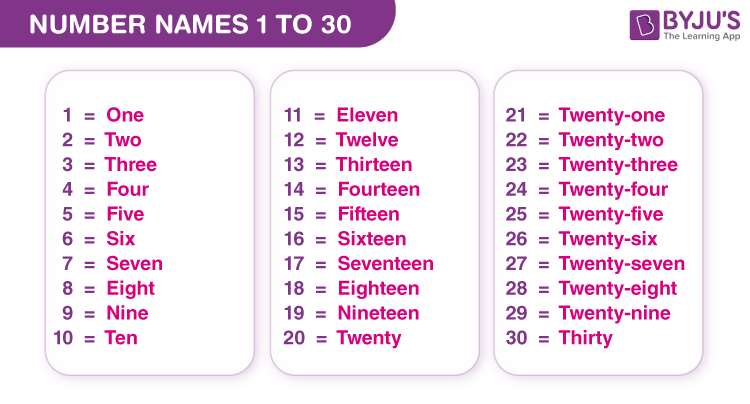Checkout JEE MAINS 2022 Question Paper Analysis : Checkout JEE MAINS 2022 Question Paper Analysis :

# Number Names 1 to 30

Number Names 1 to 30 represent the numbers 1 to 30 in word format. For example, 5 is written as five, 12 is written as twelve, etc. The number names from 1 to 30 are written using the place value such as ones, tens, hundreds, etc. In this article, we will learn the number names from 1 to 30, the rules to write number names from 1 to 30, and many solved examples in detail.

## Number Names 1 to 30 Chart

Number names 1 to 30 chart is provided here for students to learn the spellings of numbers from 1 to 30.## Numbers from 1 to 30 in Words

Number names from 1 to 30 are listed in tabular form. Go through the below table and learn the number names 1 to 30 quickly.

 Numbers from 1 to 30 Number Names from 1 to 30 1 One 2 Two 3 Three 4 Four 5 Five 6 Six 7 Seven 8 Eight 9 Nine 10 Ten 11 Eleven 12 Twelve 13 Thirteen 14 Fourteen 15 Fifteen 16 Sixteen 17 Seventeen 18 Eighteen 19 Nineteen 20 Twenty 21 Twenty-one 22 Twenty-two 23 Twenty-three 24 Twenty-four 25 Twenty-five 26 Twenty-six 27 Twenty-seven 28 Twenty-eight 29 Twenty-nine 30 Thirty

## How to Write Number Names 1 to 30?

The following rules help to write the numbers in words from 1 to 30:

• First, learn the number names from 1 to 9. i.e., one, two, three, four, five, six, seven, eight and nine.
• Learn the number names for 10 and 11. i.e., ten and eleven.
• From 12 to 19, add the suffix “teen” to the numbers in words from two to nine. For example, the number 14 is written as fourteen. i.e., Four+teen = fourteen.
• For the numbers 20 and 30, add the suffix “ty” to the number names. i.e., 20 = twenty and 30 = thirty. [Note: for counts in tens from 20 to 90, the number names always end in “ty”].
• For number names from 21 to 29, use the place value to write the numbers in words. For example, 24 is written as twenty-four. Here, 4 is in one’s place, and 2 is in ten’s place. Hence, 20+4 = 24.

### Number Names 1 to 30 Examples

Example 1:

Write the following numbers in words:

1. 12
2. 19
3. 20
4. 25

Solution:

The numbers in words are:

1. 12 – Twelve
2. 19 – Nineteen
3. 20 – Twenty
4. 25 – Twenty-five.

Example 2:

What is the value of ten plus five?

Solution:

Ten = 10

Five = 5

Ten plus five = 10+5 = 15

Hence, 15 in words is fifteen

Therefore, the value of ten plus five is fifteen.

### Number Names 1 to 30 Worksheet

1. Write the following numbers in words:

(a) 16 = ____

(b) 21 = ____

(c) 17 = ____

2. What is the value of twenty-five minus twenty?

## Frequently Asked Questions on Number Names 1 to 30

### What does the number names 1 to 30 represent?

The number names 1 to 30 represent the numeral 1 to 30 in word format.

### Write the number names from 1 to 30.

The number names from 1 to 30 are as follows: 1 – one, 2 – two, 3 – three, 4 – four, 5 – five, 6 – six, 7 – seven, 8 – eight, 9 – nine, 10 – ten, 11 – eleven, 12 – twelve, 13 – thirteen, 14 – fourteen, 15 – fifteen, 16 – sixteen, 17 – seventeen, 18 – eighteen, 19 – nineteen, 20 – twenty, 21 – twenty-one, 22 – twenty-two, 23 – twenty-three, 24 – twenty-four, 25 – twenty-five, 26 – twenty-six, 27- twenty-seven, 28 – twenty-eight, 29 – twenty-nine, 30 – thirty.

### Write the value of 9+4 in words.

The value of 9+4 is 13. Thus, the number 13 in words is thirteen.